Share

# Selina solutions for Concise Physics Class 9 ICSE chapter 7 - Reflection of Light [Latest edition]

Course
Textbook page

#### Chapters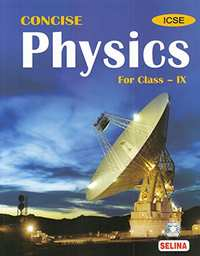## Chapter 7: Reflection of Light

Exercise - 7(A)Exercise - 7(B)Exercise - 7(C)

### Selina solutions for Concise Physics Class 9 ICSE Chapter 7 Reflection of Light Exercise - 7(A) [Pages 153 - 154]

Exercise - 7(A) | Q 1 | Page 153

What do you mean by reflection of light?

Exercise - 7(A) | Q 2 | Page 153

State which surface of a plane mirror reflects most of the light incident on it: the front smooth surface or the back silvered surface.

Exercise - 7(A) | Q 3.1 | Page 153

Explain the following term :

Plane mirror

Draw diagram/diagrams to show them.

Exercise - 7(A) | Q 3.2 | Page 153

Explain the following term:

Incident ray

Draw diagram/diagrams to show them.

Exercise - 7(A) | Q 3.3 | Page 153

Explain the following term:

Reflected ray

Draw diagram/diagrams to show them.

Exercise - 7(A) | Q 3.4 | Page 153

Explain the following term:

Angle of incidence

Draw diagram/diagrams to show them.

Exercise - 7(A) | Q 3.5 | Page 153

Explain the following term:

Angle of reflection.

Draw diagram/diagrams to show them.

Exercise - 7(A) | Q 4 | Page 153

With the help of diagrams, explain the difference between the regular and irregular reflection.

Exercise - 7(A) | Q 5 | Page 153

Differentiate between the reflection of light from a plane mirror and that from a plane sheet of paper.

Exercise - 7(A) | Q 6 | Page 153

State the two laws of reflection of light.

Exercise - 7(A) | Q 7 | Page 153

State the laws of reflection and describe an experiment to verify them .

Exercise - 7(A) | Q 8.1 | Page 153

A light ray is incident normally on a plane mirror. (a) What is its angle of incidence?

Exercise - 7(A) | Q 8.2 | Page 153

A light ray is incident normally on a plane mirror. What is the direction of reflected ray? Show it on a diagram.

Exercise - 7(A) | Q 9 | Page 153

Draw a diagram to show the reflection of a ray of light using a plane mirror. In the diagram, label the incident ray, the reflected ray, the normal, the angle of incidence and the angle of reflection.

Exercise - 7(A) | Q 10 | Page 154

Figure shows an incident ray AO and the normal ON on a plane mirror. The angle which the incident ray AO makes with mirror is 30°. (a) Find the angle of incidence. (b) Draw the reflected ray and then find the angle between the incident and reflected rays.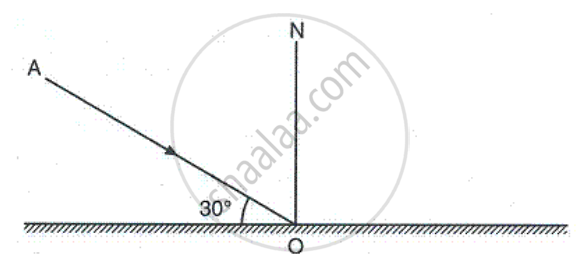Exercise - 7(A) | Q 11 | Page 154

The diagram in Figure shows a point object in front of a plane mirror MM1.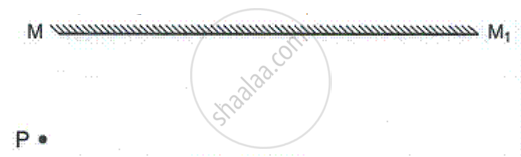(a) Complete the diagram by taking two rays from the point P to show the formation of its image.

(b) In the diagram, mark the position of eye to see the image.

(c) Is the image formed real or virtual? Explain why?

Exercise - 7(A) | Q 12 | Page 154

The diagram below in figure shows an object XY in front of a plane mirror MM1 . Draw on the diagram, path of two rays from each point X and Y of the object to show the formation of its image .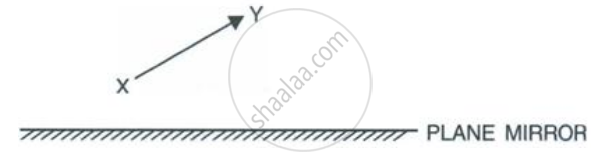Exercise - 7(A) | Q 13.1 | Page 154

Write three characteristics of the image formed by a plane mirror?

Exercise - 7(A) | Q 13.2 | Page 154

How is the position of an image related to the position of the object?

Exercise - 7(A) | Q 14 | Page 154

Differentiate between a real and a virtual image.

Exercise - 7(A) | Q 15 | Page 154

What is meant by lateral inversion of an image in a plane mirror? Explain it with the help of a ray diagram.

Exercise - 7(A) | Q 16 | Page 154

The letters on the front of an ambulance are written laterally inverted like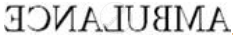. Give reason.

Exercise - 7(A) | Q 17 | Page 154

Why is it difficult to read the image of the text of a page formed due to reflection by a plane mirror?

### Selina solutions for Concise Physics Class 9 ICSE Chapter 7 Reflection of Light Exercise - 7(A) [Page 154]

Exercise - 7(A) | Q 1 | Page 154

According to the law of reflection :

• i/r = constant

• sin i /sin r = constant

• i + r = constant

• i = r

Exercise - 7(A) | Q 2 | Page 154

The image formed by a plane mirror is :

• Erect and diminished

• Erect and enlarged

• Inverted and of same size

• Erect and of same size

Exercise - 7(A) | Q 3 | Page 154

The image formed by a plane mirror is :

• real

• virtual

• virtual with lateral inversion

• real with lateral inversion

### Selina solutions for Concise Physics Class 9 ICSE Chapter 7 Reflection of Light Exercise - 7(A) [Page 154]

Exercise - 7(A) | Q 1 | Page 154

A ray is incident on a plane mirror . Its reflected ray is perpandicular to the incident ray . Find the angle of incidence .

Exercise - 7(A) | Q 2 | Page 154

A man standing in front of a plane mirror finds his image at a distance 6 metre from himself. What is the distance of man from the mirror?

Exercise - 7(A) | Q 3.1 | Page 154

An insect is sitting in front of a plane mirror at a distance 1 m from it.
(a) Where is the image of the insect formed?

Exercise - 7(A) | Q 3.2 | Page 154

An insect is sitting in front of a plane mirror at a distance 1 m from it.
(b) What is the distance between the insect and its image?

Exercise - 7(A) | Q 4 | Page 154

An object is kept at 60 cm in front of a plane mirror. If the mirror is now moved 25 cm away from the object, how does the image shift from its previous position?

Exercise - 7(A) | Q 5 | Page 154

An optician while testing the eyes of a patient keeps a chart of letters 3 m behind the patient and asks him to see the letters on the image of chart formed in a plane mirror kept at distance 2 m in front of him. At what distance is the chart seen by the patient?

### Selina solutions for Concise Physics Class 9 ICSE Chapter 7 Reflection of Light Exercise - 7(B) [Page 157]

Exercise - 7(B) | Q 1 | Page 157

Two plane mirrors are placed making an angle θ in between them. Write an expression for the number of images formed of an object placed in between the mirrors. State the condition, if any.

Exercise - 7(B) | Q 2 | Page 157

Two plane mirrors are placed making an angle θ° in between them. For an object placed in between the mirrors, if angle is gradually increased from 0° to 180°, how will the number of images change : increase, decrease or remain unchanged?

Exercise - 7(B) | Q 3 | Page 157

How many images are formed for a point object kept in between two plane mirrors at right angles to each other? Show them by drawing a ray diagram.

Exercise - 7(B) | Q 4 | Page 157

Two plane mirrors are arranged parallel and facing each other at some separation. How many images are formed for a point object kept in between them? Show the formation of images with the help of a ray diagram

Exercise - 7(B) | Q 5 | Page 157

State two uses of a plane mirror.

### Selina solutions for Concise Physics Class 9 ICSE Chapter 7 Reflection of Light Exercise - 7(B) [Page 157]

Exercise - 7(B) | Q 1 | Page 157

Two plane mirrors are placed making an angle 60° in between them. For an object placed in between the mirrors, the number of images formed will be :

• 3

• 6

• 5

• infinite

Exercise - 7(B) | Q 2 | Page 157

In the barber's shop, two plane mirrors are placed :

• Perpendicular to each other

• Parallel to each other

• At an angle 60° between them

• At angle 45° between them

### Selina solutions for Concise Physics Class 9 ICSE Chapter 7 Reflection of Light Exercise - 7(B) [Page 157]

Exercise - 7(B) | Q 1 | Page 157

State the number of images of an object placed between two mirrors, formed in each case when mirrors are inclined to each other at (a) 90°, and (b) 60°.

Exercise - 7(B) | Q 2 | Page 157

An object is placed (i) asymmetrically (ii) symmetrically, between two plane mirrors inclined at an angle of 50°. Find the number of images formed.

### Selina solutions for Concise Physics Class 9 ICSE Chapter 7 Reflection of Light Exercise - 7(C) [Pages 170 - 172]

Exercise - 7(C) | Q 1 | Page 170

What is a spherical mirror?

Exercise - 7(C) | Q 2 | Page 170

Name the two kinds of spherical mirrors and distinguish between them.

Exercise - 7(C) | Q 3 | Page 170

Define the terms pole, principal axis and centre of curvature with reference to a spherical mirror.

Exercise - 7(C) | Q 4 | Page 170

Draw suitable diagrams to illustrate the action of (i) concave mirror and (ii) convex mirror on a beam of light incident parallel to the principal axis.

Exercise - 7(C) | Q 5 | Page 170

Name the spherical mirror which (i) diverges (ii) converges the beam of light incident on it. Justify your answer by drawing a ray diagram in each case.

Exercise - 7(C) | Q 6 | Page 170

Define the terms focus and focal length of a concave mirror. Draw diagram to illustrate your answer.

Exercise - 7(C) | Q 7 | Page 170

Explain the meaning of the terms focus and focal length in case of a convex mirror, with the help of suitable ray diagram.

Exercise - 7(C) | Q 8 | Page 170

State the direction of incident ray which after reflection from a spherical mirror retraces its path. Give a reason to your answer.

Exercise - 7(C) | Q 9.1 | Page 170

Name the mirrors shown in Figure (a) and (b).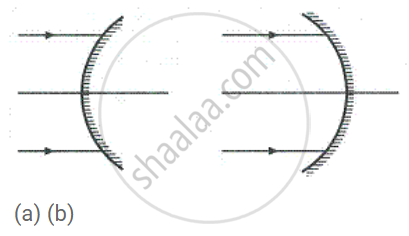Exercise - 7(C) | Q 9.2 | Page 170

In each case (a) and (b), draw reflected rays for the given incident rays and mark focus by the symbol F.Exercise - 7(C) | Q 10 | Page 170

Complete the following diagrams in Figure by drawing the reflected rays for the incident rays 1 and 2.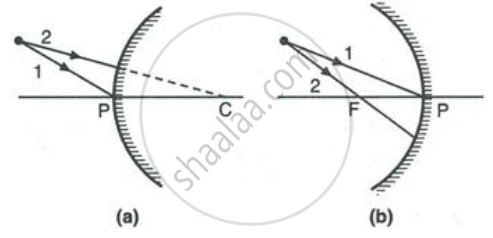Exercise - 7(C) | Q 11 | Page 171

Complete the following diagrams shown in Fig. by drawing the reflected ray for each of the incident ray and B.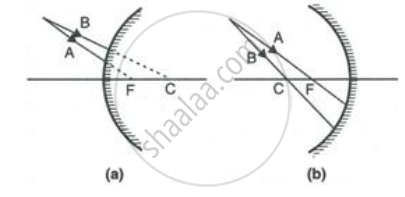Exercise - 7(C) | Q 12 | Page 171

State the two convenient rays that are chosen to construct the image by a spherical mirror for a given object? Explain your answer with the help of suitable ray diagrams.

Exercise - 7(C) | Q 13 | Page 171

Figure shows a concave mirror with its pole at P, focus F and centre of curvature C. Draw ray diagram to show the formation of image of an object OA.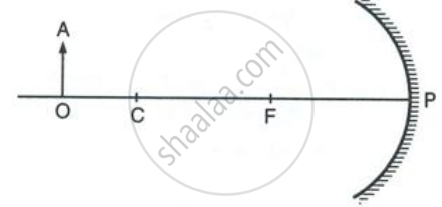Exercise - 7(C) | Q 14 | Page 171

Figure shows a concave mirror with its pole at P, focus and centre of curvature C. Draw ray diagram to show the formation of image of an object OA.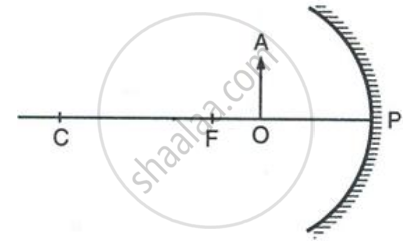Exercise - 7(C) | Q 15 | Page 171

The diagram below in Figure, shows a convex mirror. C is its centre of curvature and F is its focus. (i) Draw two rays from A and hence locate the position of image of object OA. Label the image IB. (ii) State three characteristics of the image.

Exercise - 7(C) | Q 16 | Page 171

Draw a ray diagram to show the formation of image by a concave mirror for an object placed between its pole and focus. State three characteristics of the image.

Exercise - 7(C) | Q 17 | Page 171

Draw a ray diagram to show the formation of image by a concave mirror for the object beyond its centre of curvature. State three characteristics of the image.

Exercise - 7(C) | Q 18 | Page 171

Draw a ray diagram to show the formation of image of an object kept in front of a convex mirror. State three characteristics of the image.

Exercise - 7(C) | Q 19 | Page 171

Name the mirror which always produces an erect and virtual image. How is the size of image related to the size of object?

Exercise - 7(C) | Q 20.1 | Page 171

For what position of object, the image formed by a concave mirror is magnified and erect?

Exercise - 7(C) | Q 20.2 | Page 171

State whether the image in part (a) is real or virtual?

Exercise - 7(C) | Q 21.1 | Page 171

State the position of object for which the image formed by a concave mirror is of same size.

Exercise - 7(C) | Q 21.2 | Page 171

Write two more characteristics of the image.

Exercise - 7(C) | Q 22.1 | Page 171

What is a real image?

Exercise - 7(C) | Q 22.2 | Page 171

What type of mirror can be used to obtain a real image of an object?

Exercise - 7(C) | Q 22.3 | Page 171

Does the mirror mentioned in part (b) form real image for all locations of the object?

Exercise - 7(C) | Q 23 | Page 171

Discuss the position and nature of image formed by a concave mirror when an object is moved from infinity towards the pole of mirror.

Exercise - 7(C) | Q 24 | Page 171

Discuss the position and nature of image formed by a convex mirror when an object is moved from infinity towards the pole of mirror.

Exercise - 7(C) | Q 25.1 | Page 171

Name the kind of mirror used to obtain :

A real and enlarged image

Exercise - 7(C) | Q 25.2 | Page 171

Name the kind of mirror used to obtain:

A virtual and enlarged image

Exercise - 7(C) | Q 25.3 | Page 171

Name the kind of mirror used to obtain:

A virtual and diminished image

Exercise - 7(C) | Q 25.4 | Page 171

Name the kind of mirror used to obtain:

A real and diminished image.

Exercise - 7(C) | Q 26 | Page 171

How is the focal length of a spherical mirror related to its radius of curvature?

Exercise - 7(C) | Q 27 | Page 171

Write the spherical mirror's formula and explain the meaning of each symbol used in it.

Exercise - 7(C) | Q 28 | Page 172

What is meant by magnification? Write its expression. What is its sign for the (a) real (b) virtual, image?

Exercise - 7(C) | Q 29 | Page 172

Upto what maximum distance from the pole the image in a convex mirror can be obtained? what will be the location of object then ?

Exercise - 7(C) | Q 30 | Page 172

Upto what maximum distance from a concave mirror, the image can be obtained? What will be the location of object for it?

Exercise - 7(C) | Q 31 | Page 172

How will you distinguish between a plane mirror, a concave mirror and a convex mirror, without touching them?

Exercise - 7(C) | Q 32 | Page 172

State two uses of a concave mirror.

Exercise - 7(C) | Q 33 | Page 172

State the kind of mirror used

(a) By a dentist,

(b) As a search-light reflector.

Exercise - 7(C) | Q 34 | Page 172

(a) When a concave mirror is used as a shaving mirror, where is the person's face in relation to the focus of mirror?

(b) State three characteristics of the image seen in part (a).

Exercise - 7(C) | Q 35 | Page 172

Which mirror will you prefer to use as a rear view mirror in a car : plane mirror or convex mirror? Give one reason.

Exercise - 7(C) | Q 36 | Page 172

Why does a driver use a convex mirror instead of a plane mirror as a rear view mirror?

### Selina solutions for Concise Physics Class 9 ICSE Chapter 7 Reflection of Light Exercise - 7(C) [Page 172]

Exercise - 7(C) | Q 1 | Page 172

For an incident ray directed towards centre of curvature of a spherical mirror the reflected ray:

• Retraces its path

• Passes through the focus

• Passes through the pole

• Becomes parallel to the principal axis.

Exercise - 7(C) | Q 2 | Page 172

The image formed by a convex mirror is

• Erect and diminished.

• Erect and enlarged.

• Inverted and diminished.

• Inverted and enlarged.

Exercise - 7(C) | Q 3 | Page 172

A real and enlarged image can be obtained by using a

•  Convex mirror

• Plane mirror

• Concave mirror

• Either convex or plane mirror.

### Selina solutions for Concise Physics Class 9 ICSE Chapter 7 Reflection of Light Exercise - 7(C) [Page 172]

Exercise - 7(C) | Q 1 | Page 172

The radius of curvature of a convex mirror is 40 cm. Find its focal length.

Exercise - 7(C) | Q 2 | Page 172

The focal length of a concave mirror is 10 cm. Find its radius of curvature.

Exercise - 7(C) | Q 3 | Page 172

An object of height 2 cm is placed at a distance 20 cm in front of a concave mirror of focal length 12 cm. Find the position, size and nature of the image .

Exercise - 7(C) | Q 4 | Page 172

An object is placed at 4 cm distance in front of a concave mirror of radius of curvature 24 cm. Find the position of image. Is the image magnified?

Exercise - 7(C) | Q 5 | Page 172

At what distance from a concave mirror of focal length 25 cm should an object be placed so that the size of image is equal to the size of the object.

Exercise - 7(C) | Q 6 | Page 172

An object 5 cm high is placed at a distance 60 cm in front of a concave mirror of focal length 10 cm. Find the position and size of the image.

Exercise - 7(C) | Q 7 | Page 172

A point light source is kept in front of a convex mirror at a distance of 40 cm. The focal length of the mirror is 40 cm. Find the position of image by drawing.

Exercise - 7(C) | Q 8 | Page 172

When an object of height 1 cm is kept at a distance 4 cm from a concave mirror, its erect image of height 1.5 cm is formed at a distance 6 cm behind the mirror. Find the focal length of mirror, by drawing.

Exercise - 7(C) | Q 9 | Page 172

An object of length 4 cm is placed in front of a concave mirror at distance 30 cm. The focal length of mirror is 15 cm.

1. Where will the image form?
2. What will be the length of image?
Exercise - 7(C) | Q 10 | Page 172

A concave mirror forms a real image of an object placed in front of it at a distance 30 cm, of size three times the size of object. Find (a) the focal length of mirror (b) position of image.

Exercise - 7(C) | Q 11 | Page 172

A concave mirror forms a virtual image of size twice that of the object placed at a distance 5 cm from it.

Find : (a) the focal length of the mirror (b) position of image

Exercise - 7(C) | Q 12 | Page 172

The image formed by a convex mirror is of size one third the size of object. How are u and v related?

Exercise - 7(C) | Q 13 | Page 172

The erect image formed by a concave mirror is of size double the size of object. How are u and v related?

Exercise - 7(C) | Q 14 | Page 172

The magnification for a mirror is -3. How are u and v related?

## Chapter 7: Reflection of Light

Exercise - 7(A)Exercise - 7(B)Exercise - 7(C)## Selina solutions for Concise Physics Class 9 ICSE chapter 7 - Reflection of Light

Selina solutions for Concise Physics Class 9 ICSE chapter 7 (Reflection of Light) include all questions with solution and detail explanation. This will clear students doubts about any question and improve application skills while preparing for board exams. The detailed, step-by-step solutions will help you understand the concepts better and clear your confusions, if any. Shaalaa.com has the CISCE Concise Physics Class 9 ICSE solutions in a manner that help students grasp basic concepts better and faster.

Further, we at Shaalaa.com provide such solutions so that students can prepare for written exams. Selina textbook solutions can be a core help for self-study and acts as a perfect self-help guidance for students.

Concepts covered in Concise Physics Class 9 ICSE chapter 7 Reflection of Light are Reflection of Light, Images Formed by a Pair of Parallel and Perpendicular Plane Mirrors, Laws of Reflection, Spherical Mirrors, Uses of Spherical Mirrors, Concept of Reflection, Plane Mirror - Characteristics of the Image Formed (Lateral Inversion, Same Size, Distance is Preserved)., Concept of Magnifying Glass.

Using Selina Class 9 solutions Reflection of Light exercise by students are an easy way to prepare for the exams, as they involve solutions arranged chapter-wise also page wise. The questions involved in Selina Solutions are important questions that can be asked in the final exam. Maximum students of CISCE Class 9 prefer Selina Textbook Solutions to score more in exam.

Get the free view of chapter 7 Reflection of Light Class 9 extra questions for Concise Physics Class 9 ICSE and can use Shaalaa.com to keep it handy for your exam preparation